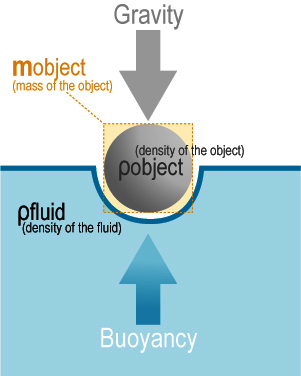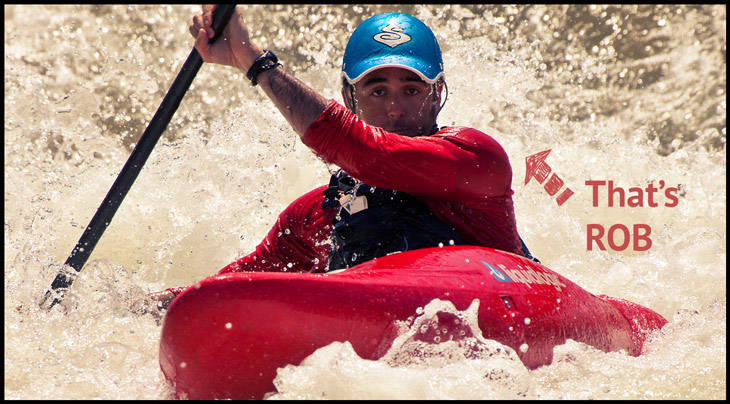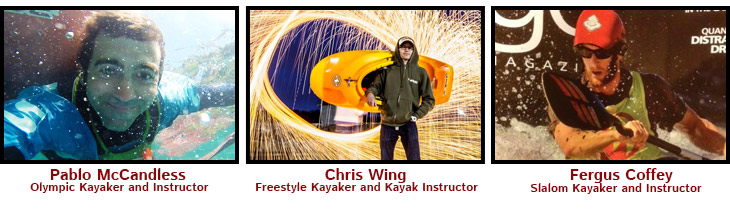# Buoyancy Science

If you swim, kayak, or take a bath, you’ll definitely want to understand the science of “Buoyancy,” by watching this video. But don’t stop there. Get all your friends to wade into the topic by sharing it in your classroom with a combination of watching the video then collectively taking part in a discussion and the Lesson Guide below that really dives into the practical science of investigating Buoyancy. What? You’re not in school anymore? That’s no reason to stop learning cool science about sports. Explore the lesson stuff anyway… and read on.

### Here’s the background to get you started.

In science, buoyancy is an upward force exerted by a fluid that opposes the weight and size of an immersed object. Here’s the formula for buoyancy of an object in the ocean… F = (Vw)  F = force, V = volume of fluid displaced in ft3,  w = specific weight of sea water, 64lbs/ft3

Back in 212 B.C., the old physics wizard Archimedes figured out that… “Any object, wholly or partially immersed in a fluid, is buoyed up by a force equal to the weight of the fluid displaced by the object.” Well, he was mostly right. He left out an important part about the weight of the displaced fluid is directly proportional to the volume of the displaced fluid. The simple concept is that the buoyancy force on any object is  equal to the weight of the fluid displaced by the object – and the displaced fluid is affected by the volume of the object. So that means with submerged objects with equal masses, the objects with greater volume will have greater buoyancy. This is also known as upthrust. That all makes sense, right? Thirty pounds of plastic shaped into a bowling ball will sink. But reshape that 30 pounds of plastic into a kayak and you have extra buoyancy to spare – enough to also float a person paddling. Why? It’s in the volume of the weight which affects the volume of displaced fluid.So an object with a density greater than that of the fluid in which it is submerged tends to sink. If the object is either less dense than the liquid or is shaped appropriately (like our kayak), the force can keep the object afloat.

Here’s the formula for,  Buoyancy Force (B) = ρ V g

• Where, ρ = Density of Fluid.
• V = Displaced Volume.
• g = 9.8 (gravity).

Okay, enough with all the formulas. Let’s have some serious fun by taking all this buoyancy learning to the next level. Here’s what to do;

• Have the entire classroom watch the video to get the concept of buoyancy
• Have your teacher download the free Lesson Guides below so the entire class can explore buoyancy with some practical hands-on experiments.

Also, here are some helpful links to buoyancy experiments and learning tools. Have fun but don’t sink!